Processing ACA Monitor Window DataOverview

Synopsis:

The Aspect Camera Assembly (ACA) is capable of providing simultaneous optical monitoring of a target during an observation. This thread describes the steps involved in processing the ACA monitor data.

Purpose:

Generate a photometric light curve for a Chandra target which was observed using an ACA monitor window. This is accomplished by use of the monitor_photom script.

Last Update: 09 Dec 2019 - Updated for matplotlib 3.1

Background Information

This thread assumes familiarity with the basic ACA operating principles as described in the Pointing Control and Aspect Determination System chapter of the Proposers' Observatory Guide (POG). This includes the concepts of image slots and readout windows.

The key challenge in deriving a photometric light curve from ACA monitor window data is determining the background dark current. Due to ionizing radiation in the space environment, more than 1/3 of the pixels on the ACA CCD have detectable damage as manifested by an elevated dark current, as shown in Figure 1.

Figure 1: Dark Current Histogram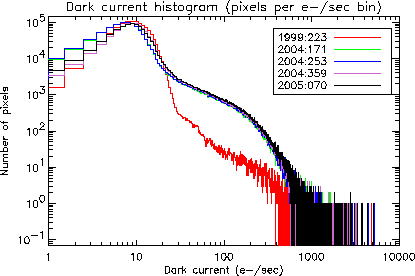Figure 1: Dark Current Histogram

The main Gaussian peak of the dark current distribution, representing undamaged pixels, dominates below about 30 e-/sec. Above that value the pixels have been damaged by cosmic radiation. In aspect pipeline processing a pixel with a dark current of more than 200 e-/sec is considered "warm" because at this level centroiding can be perturbed. A 15th magnitude source produces only about 500 e-/sec, so for faint sources the impact of these warm pixels is significant. To make matters worse, many warm pixels show time dependent flickering behavior in which the pixel dark current can abruptly change by a factor of two or more. This flickering occurs on time scales of thousands of seconds, making it essentially impossible to produce a reliable dark current map using the ACA calibration mode. Instead we use on-the-fly warm pixel detection.

The basic idea of the detection algorithm is to sample pixels from the outer edge of the 8x8 pixel readout window and look for values higher than a threshold. At the edge the contamination of the dark current measurement from the monitor star is minimized. Since the readout window moves with respect to the CCD due to dither, the edge pixels end up sampling much of the CCD region used for imaging. In this way one can build up a map of warm pixels.

There are three limitations to the algorithm:

• Background pixel sampling is not complete nor uniform.

• For brighter stars even the edge pixels have significant contribution from the star light so it is not possible to get a true dark current measurement. The dark_ratio tool parameter (see below) specifies that the warm pixel threshold be no less than dark_ratio * avg_source_counts.

• The current algorithm in the monitor_photom script does not account for flickering, though this could be done with some effort.

Get Started

Only monitor window data processed with an ASCDSVER of DS 7.6.0 or higher should be used in this thread.

Note that monitor window data are only available if the observer has specified target photometry (Photometry=Y) in the observation setup. This can be determined by examining the Obscat parameters, available via Web Chaser for a specific ObsID.

Obtaining the ACA image data

Or they can be retrieved via WebChaser by selecting the Secondary data products.

These files contain the processed ACA telemetry and image data for the observation. The "7" in "adat71" refers to image slot 7, which is the slot where monitor window data always appear. While there may be many more files in the data package (i.e. adat01 - adat61), this thread will only be using the adat71.fits data.

The key data columns in this file are:

Name Unit Datatype Description
time sec Real8 Time at start of integration
aca_comp_bkg_avg count Real4 Image background level (e-)
img_raw[8,8] adu Real4(8x8) Raw image from ACA
img_corr[8,8] count Real4(8x8) Calibrated image (e-)
fit_resid[8,8] count Real4(8x8) Gaussian fit residuals (e-)
img_row0 pixel Int2 Row position of lower left pixel
img_col0 pixel Int2 Column position of lower left pixel
img_excl[8,8]   Byte(8x8) Exclude pixel flag

Merge the Data Files (if necessary)

Typically the image data are split over a number of files, so the first step is to merge the ACA image data into a single file using dmmerge. This observation only has a single file so no merging is necessary. The steps below are shown as reference for other datasets that have multiple files:

The ACA files are input to the tool as a stack; see ahelp stack for more information.

Run the monitor_photom script

The main processing steps of the monitor_photom script are:

• Read the image data file.

• Median filter image data in time on a pixel-by-pixel basis to remove cosmic rays.

• Search for "warm" pixels that have a dark current well outside the normal distribution. These pixels can significantly affect photometry as they dither in and out of the 8x8 pixel image readout window.

• Subtract the background from each image readout. For warm pixels the detected value for that pixel is used, while for all others the median background dark current reported by the ACA is used.

• Produce light curves in counts, counts/sec, and mags.

This script has a parameter file associated with it:

unix% plist monitor_photom

Parameters for /home/user/cxcds_param4/monitor_photom.par

infile =                  ACA image data file
outfile =                  Output light curve
(dark_ratio = 0.005)           Dark ratio
(min_dark_limit = 80.0)            Minimum warm pixel dark current
(min_dark_meas = 10)              Minimum warm pixel measurements
(max_dither_motion = 10)              Maximum possible dither motion (pixels)
(verbose = 0)               Amount of tool chatter
(clobber = no)              Remove output file if it already exists?
(mode = ql)

The parameters dark_ratio, min_dark_limit, and min_dark_meas affect the way in which warm pixels are detected. The default values in the supplied parameter file are a good starting place, but it is often helpful to adjust the the dark_ratio and/or min_dark_limit to obtain better results.

monitor_photom
outfile = monitor_lc.fits
dark_ratio = 0.005
min_dark_limit = 80
min_dark_meas = 10
max_dither_motion = 10
verbose = 1
clobber = no
mode = ql

Filtering image data (cosmic ray removal)...
Stacking dark current data...
Average counts  (e-) = 29882.9175202
Warm dark limit (e-) = 149.414587601
Warm pixel at CCD (row,col) = (-6,8)	 Dark current (e-) = 390.0
Warm pixel at CCD (row,col) = (-13,9)	 Dark current (e-) = 320.0
Warm pixel at CCD (row,col) = (-7,10)	 Dark current (e-) = 160.0
Warm pixel at CCD (row,col) = (-11,11)	 Dark current (e-) = 215.0
Warm pixel at CCD (row,col) = (-7,11)	 Dark current (e-) = 280.0
Warm pixel at CCD (row,col) = (-5,13)	 Dark current (e-) = 345.0
Warm pixel at CCD (row,col) = (-6,16)	 Dark current (e-) = 355.0
Warm pixel at CCD (row,col) = (-9,17)	 Dark current (e-) = 185.0
Warm pixel at CCD (row,col) = (-9,18)	 Dark current (e-) = 215.0

The script does cosmic ray removal, reports on the average image counts (in e- for the 1.696 second integration) and the minimum dark current for warm pixels, and then makes a dark current stack to detect warm pixels.

The light curve is written to the specified FITS file (monitor_lc.fits). It contains columns with the time, counts, count rate, magnitude, and background-subtracted image. The magnitude is defined as

$m_{ACA} = 10.32 - 2.5 * log_{10}\left( \frac{cnt\_rate}{5263.0} \right)$

An approximate formula relating B and V magnitude to mACA is given in the POG:

$m_{ACA} = V + 0.426 - 1.06*(B-V) + 0.617*(B-V)^2 - 0.307*(B-V)^3$

This is based on the typical spectral energy distribution of bright main sequence stars.

Examine the Results

A series of python commands are used as a first step in examining the results from the script:

import matplotlib.pylab as plt

mag = lc.get_column("mag").values
toff = lc.get_column("time").values
toff = (toff-toff)/1000.0

plt.subplots(2, 1, sharex='col')
plt.subplot(2,1,1)

plt.plot(toff,mag, marker="None")
plt.ylabel(r"Image magnitude ($\mathregular{m_{ACA}}$)")
plt.title("ObsID 11022 Optical Monitor Data")
plt.gca().invert_yaxis()

plt.subplot(2,1,2)
counts =  lc.get_column("count_rate").values
plt.plot(toff, counts, marker="None")
plt.ylabel(r"Image count rate ($\mathregular{e^-/sec}$)")
plt.xlabel("Time from Obs Start (ksec)")

The plot results are shown in Figure 2

Figure 2: ACA Monitor Data: magnitude and count rate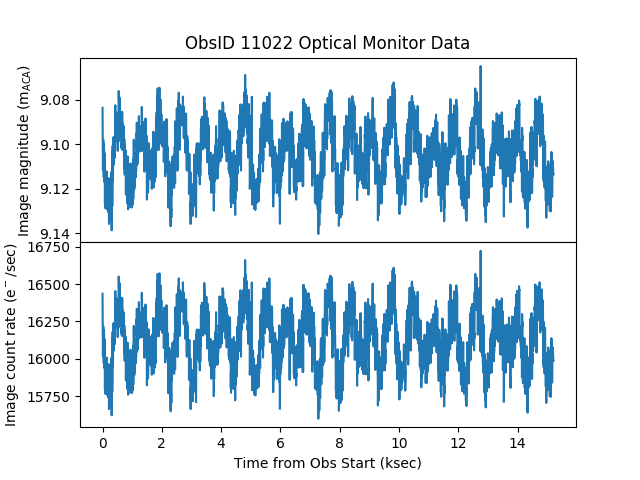Figure 2: ACA Monitor Data: magnitude and count rate

The data clearly shows a periodicity with an amplitude of approximately 1000 e-/sec. To check whether the periodicity is the result of the star dithering across an unidentified warm pixel, the data can be period folded at the telescope dither frequencies. The default dither frequencies for ACIS and HRC are listed below (taken from the the Chandra Proposer's Guide).

Standard Chandra dither frequencies
Direction ACIS HRC
Pitch 707.1 sec 768.6 sec
Yaw 1000.0 sec 1087.0 sec

These values are standard for most observations. Users can check for non-standard dither frequencies by checking the Details page of Web Chaser. Users can check which is the dominant frequency by computing the power spectrum of the light curve. In the example below, the mean counts are subtracted off each light curve bin to remove the 0-frequency spike in the power spectrum.

unix% dmstat "monitor_lc.fits[cols counts]"
counts
min:	26455 	      @:	1776
max:	28362.5 	      @:	3111
mean:	27347.435057
sigma:	314.80082893
sum:	101458984.06
good:	3710
null:	0

unix% dmtcalc monitor_lc.fits lc0.fits expr='c0=counts-27347.435057'
unix% apowerspectrum lc0.fits'[cols time,c0]' none powerspec.fits crop=yes clob+

Since the data are entirely real valued, the power spectrum is symmetric about the Nyquist frequency so the data can be crop'ed. The power spectrum can now be plotted and the expected frequencies identified

import matplotlib.pyplot as plt

freq = psout.get_column("frequency").values
pwr = psout.get_column("data").values

plt.plot(freq,pwr, marker="None", linewidth=2, color="black")
plt.xscale("log")
plt.yscale("log")
plt.ylim(bottom=100)
plt.axvline( 1.0/707.1, color="red")
plt.axvline( 1.0/1000.0, color="blue")
plt.xlabel(r"Frequency [$\mathregular{s^{-1}}$]")
plt.ylabel("Power")
plt.title("Power Spectrum of Count Data")

As shown in Figure 3, there are two peaks in the power spectrum that correspond exactly to the two dither frequencies, confirming that the periodicity is induced by dither.

Figure 3: Power Spectrum of ACA Optical Monitor light curve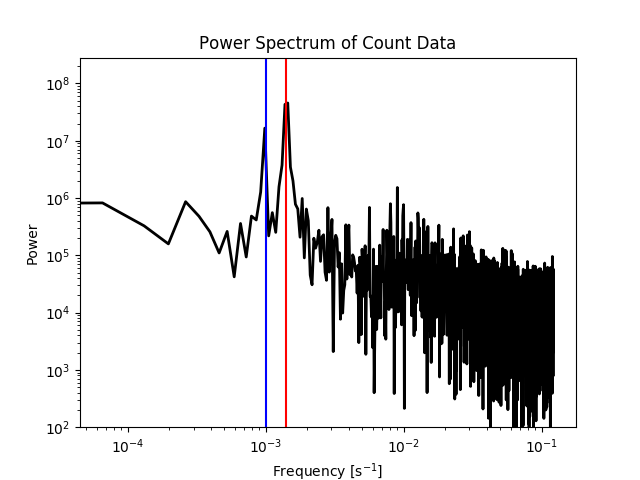Figure 3: Power Spectrum of ACA Optical Monitor light curve

The power spectrum of the ACA optical monitor data shows two spikes. The red line is at a period of 707.1 sec and the blue line is at a period of 1000.0 sec; both are the standard dither frequencies.

The light curve data can now be folded at the 707.1 sec dither frequency. This is done using the NumPy mod (modulo) operation.

import matplotlib.pylab as plt
import numpy as np

tt = tab.get_column("time").values
cc = tab.get_column("counts").values
fold = np.mod( tt, 707.1 )

plt.plot(fold,cc,marker="o",linestyle="None",fillstyle="none")
plt.xlabel("Time modulo 707.1 [sec]")
plt.ylabel("Counts [e-]")
plt.title("Period Folded Light Curve for ObsId 11022")

The data after being folded are shown in Figure 4. The sinusoidal pattern is the indication that the period chosen to fold the data was correct.

Figure 4: Period Folded Light Curve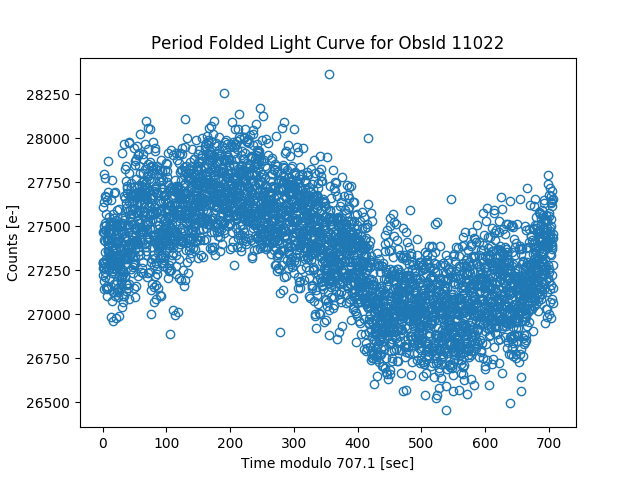Figure 4: Period Folded Light Curve

The light curve data after 707.1 sec period folding. The sinusoidal nature of the data becomes very clear. The 707.1 sec period was chosen since it was the frequency with most power as shown in Figure 3.

Problem Case: Lost faint star track

There are situations when the ACA cannot track the optical monitor star. When that happens the utility of the optical monitor data becomes limited. One such example is OBS_ID 4924.

When the monitor_photom script is run on the merged pcad_adat71.fits files, the results are shown in Figure 5

Figure 5: ACA Monitor Data for Problematic OBS_ID 4924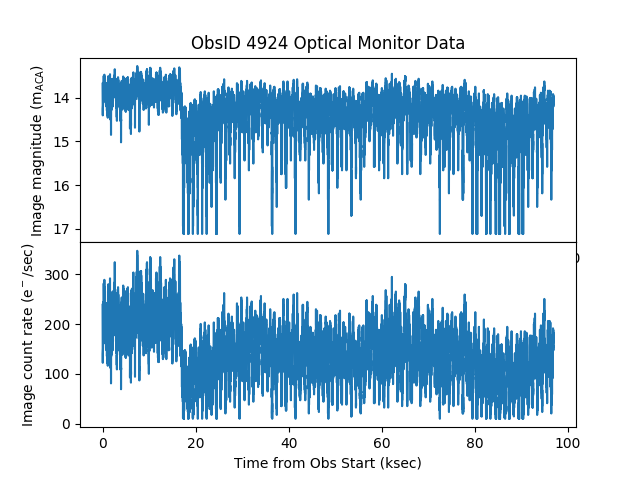Figure 5: ACA Monitor Data for Problematic OBS_ID 4924

There is an obvious jump around 17 ksec into the observation which is due to a limitation of the ACA flight software. Because the source in a monitor window may not be bright enough for the ACA to independently track, the ACA relies on the motion of another "designated track star" to move the monitor window in lock step. However, if this designated track star is lost by the ACA, even momentarily, then the ACA stops moving the monitor window and leaves it fixed at its last position on the CCD. Instead of the monitor window tracking the motion of the source as Chandra dithers, the source now dithers within the fixed window, possibly going completely outside the readout window. This can be explicitly seen via manipulation of the image data file:

import matplotlib.pylab as plt

toff = dat.get_column("time").values
row = dat.get_column("img_row0").values
col = dat.get_column("img_col0").values
toff = (toff-toff)/1000.0

plt.subplots(2, 1, sharex='col')
plt.subplot(2,1,1)

plt.plot(toff,row, marker="None")
plt.title("ObsID 4924 Optical Monitor Data")
plt.xlim(12,22)

plt.subplot(2,1,2)
plt.plot(toff,col, marker="None")
plt.xlabel("Time from Obs Start (ksec)")
plt.xlim(12,22)

These commands result in Figure 6

Figure 6: ACA Monitor Window readout coordinates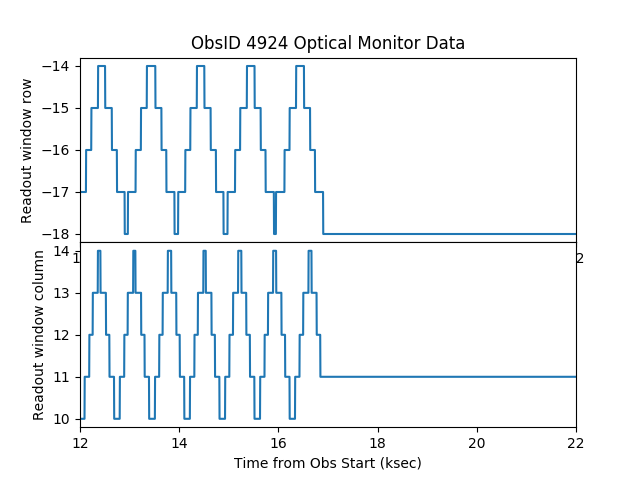Figure 6: ACA Monitor Window readout coordinates

The impact to the photometry due to not tracking the source can be seen in Figure 7.

Figure 7: ACA Monitor Data: magnitude and count rate (zoom)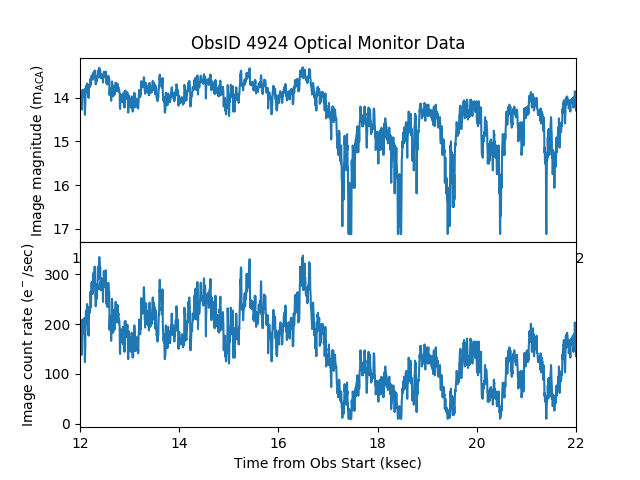Figure 7: ACA Monitor Data: magnitude and count rate (zoom)

The dither pattern is no longer due to the source dithering across warm pixels, but instead is due to the stationary ACA monitor window dithering onto the source. The data after the loss of tracking is not generally useful.